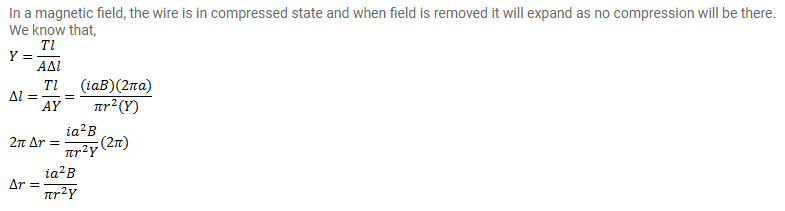# Suppose that the radius of cross-section of the wire used

Question:

Suppose that the radius of cross-section of the wire used in the previous problem is $r$. Find the increase in the radius of the loop if the magnetic field is switched off. The Young's modulus of the material of the wire is $Y$.

Solution: